Ex 7.1

Chapter 7 Class 10 Coordinate Geometry
Serial order wise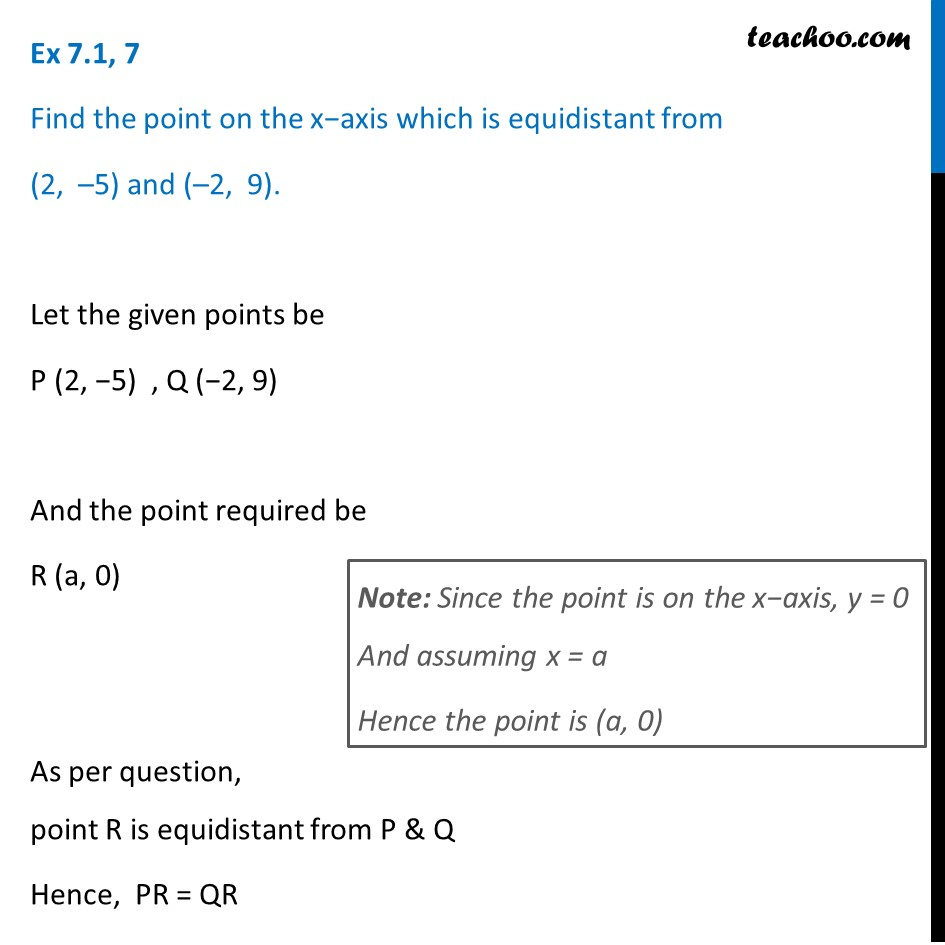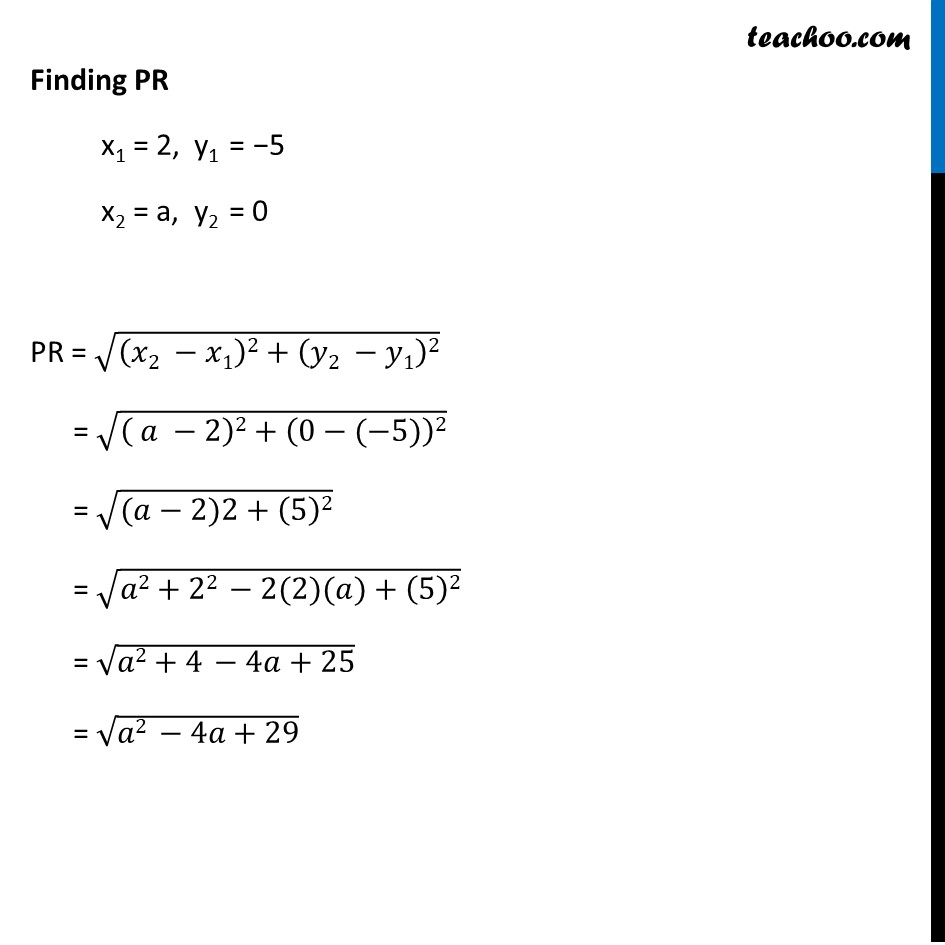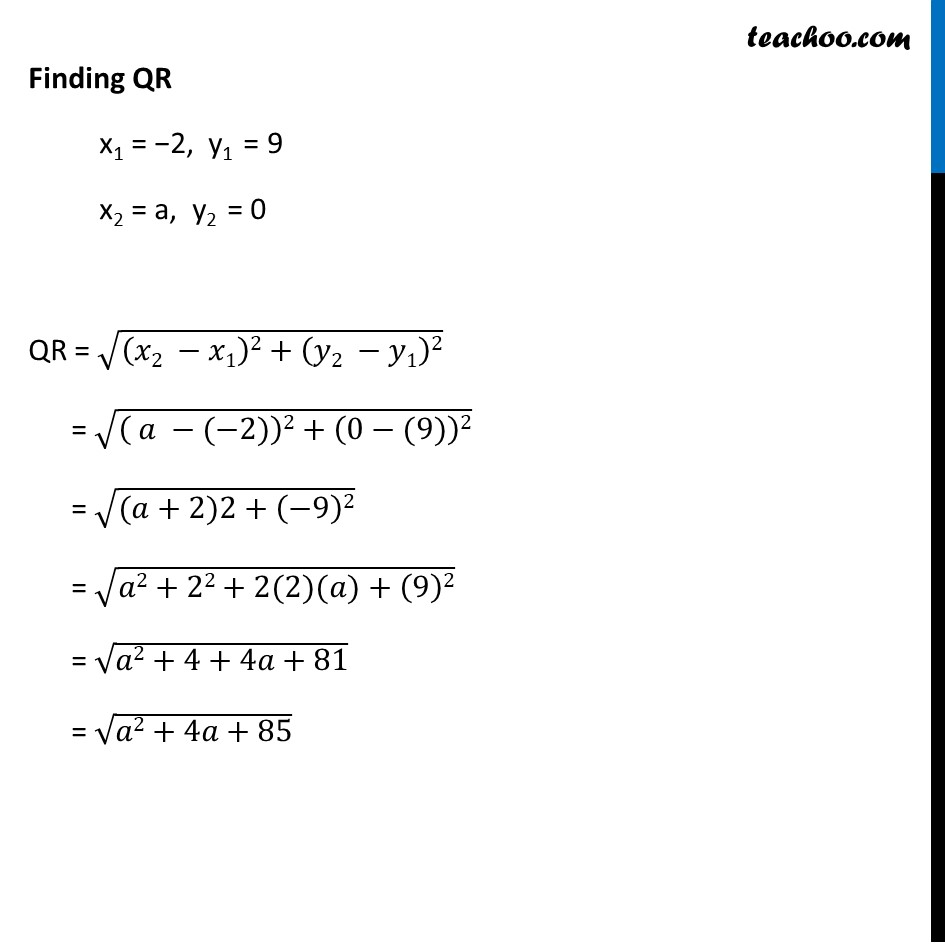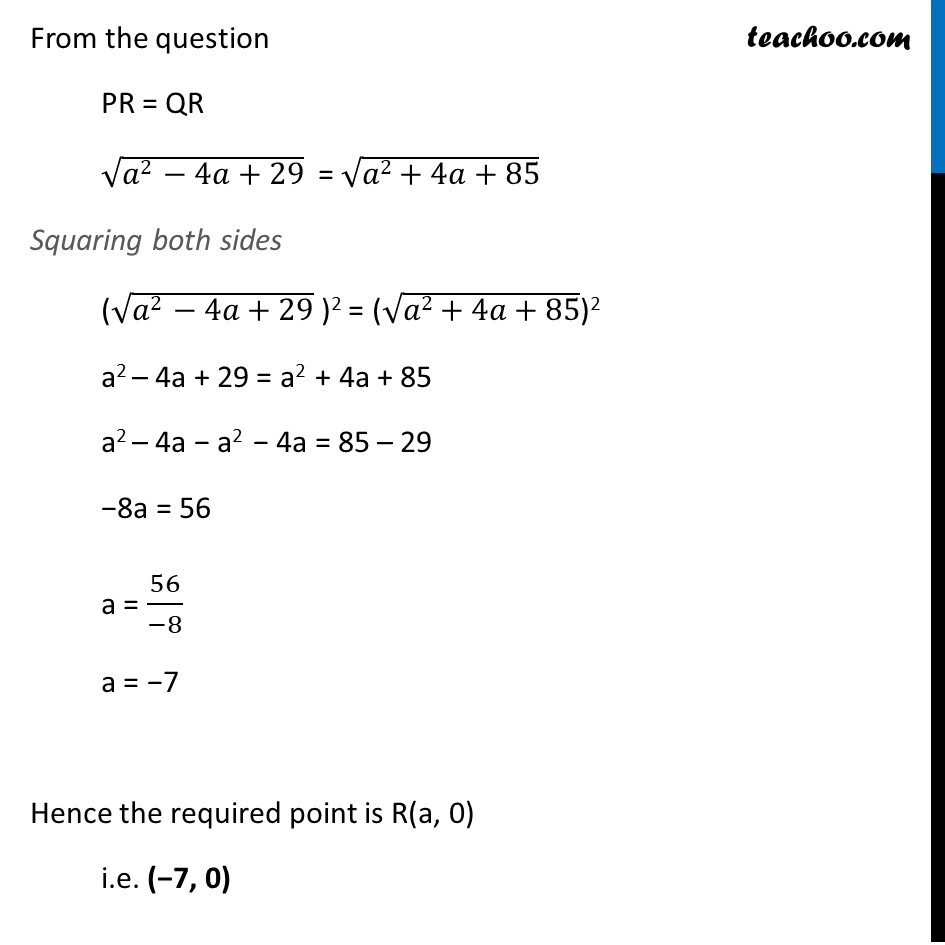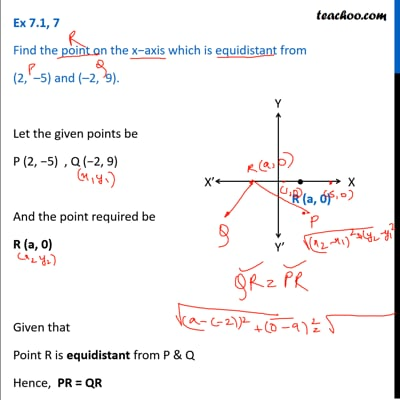This video is only available for Teachoo black users

### Transcript

Ex 7.1, 7 Find the point on the x−axis which is equidistant from (2, –5) and (–2, 9). Let the given points be P (2, −5) , Q (−2, 9) And the point required be R (a, 0) As per question, point R is equidistant from P & Q Hence, PR = QR Note: Since the point is on the x−axis, y = 0 And assuming x = a Hence the point is (a, 0) Finding PR x1 = 2, y1 = −5 x2 = a, y2 = 0 PR = √((𝑥2 −𝑥1)2+(𝑦2 −𝑦1)2) = √(( 𝑎 −2)2+(0−(−5))2) = √((𝑎−2)2+(5)2) = √(𝑎2+22 −2(2)(𝑎)+(5)2) = √(𝑎2+4 −4𝑎+25) = √(𝑎2 −4𝑎+29) Finding QR x1 = −2, y1 = 9 x2 = a, y2 = 0 QR = √((𝑥2 −𝑥1)2+(𝑦2 −𝑦1)2) = √(( 𝑎 −(−2))2+(0−(9))2) = √((𝑎+2)2+(−9)2) = √(𝑎2+22+2(2)(𝑎)+(9)2) = √(𝑎2+4+4𝑎+81) = √(𝑎2+4𝑎+85) From the question PR = QR √(𝑎2 −4𝑎+29) = √(𝑎2+4𝑎+85) Squaring both sides (√(𝑎2 −4𝑎+29) )2 = (√(𝑎2+4𝑎+85))2 a2 – 4a + 29 = a2 + 4a + 85 a2 – 4a − a2 − 4a = 85 – 29 −8a = 56 a = 56/(−8) a = −7 Hence the required point is R(a, 0) i.e. (−7, 0)